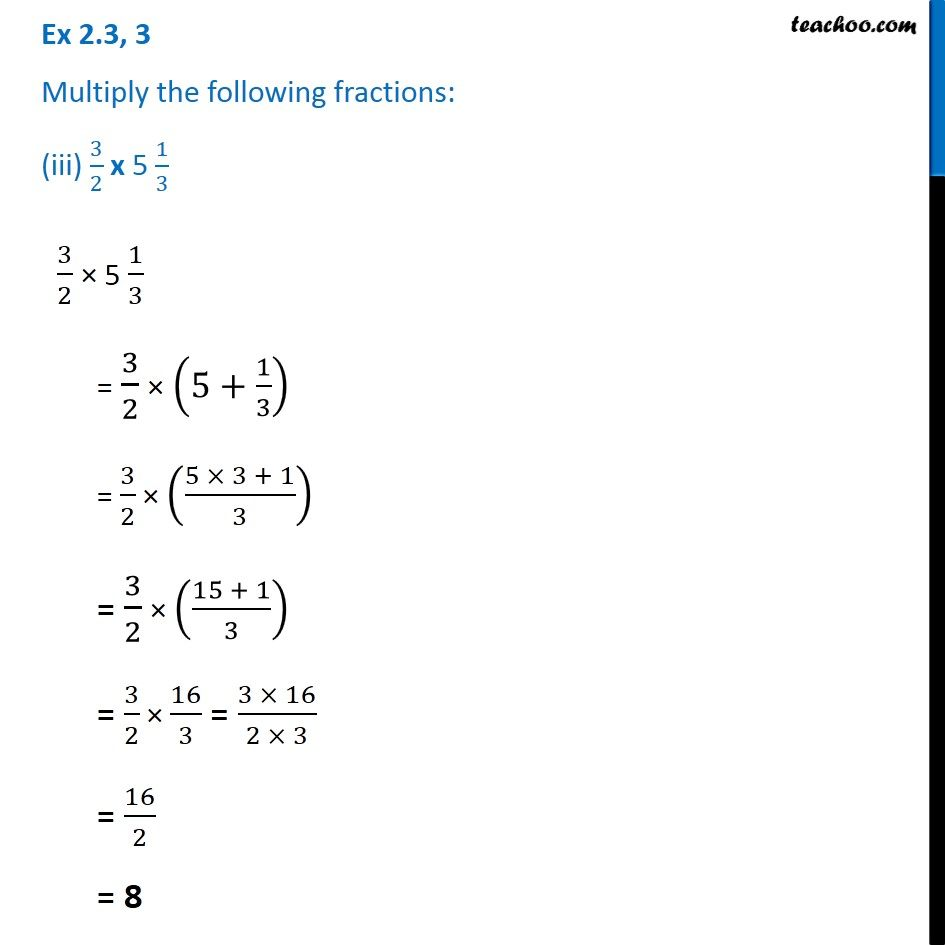Ex 2.2

Chapter 2 Class 7 Fractions and Decimals
Serial order wiseLearn in your speed, with individual attention - Teachoo Maths 1-on-1 Class

### Transcript

Ex 2.2, 3 Multiply the following fractions: (iii) 3/2 x 5 1/3 3/2 × 5 1/3 = 3/2 × (5+1/3) = 3/2 × ((5 × 3 + 1)/3) = 3/2 × ((15 + 1)/3) = 3/2 × 16/3 = (3 × 16)/(2 × 3) = 16/2 = 8